STXXL  1.4-dev
stxxl::sort -- Sorting Comparison-Based

stxxl::sort is an external memory equivalent to STL std::sort. The design and implementation of the algorithm is described in detail in .

# Prototype

template < typename ExtIterator,
typename StrictWeakOrdering
>
void sort ( ExtIterator first,
ExtIterator last,
StrictWeakOrdering cmp,
)

# Description

stxxl::sort sorts the elements in [first, last) into ascending order, meaning that if i and j are any two valid iterators in [first, last) such that i precedes j, then *j is not less than *i. Note: as std::sort, stxxl::sort is not guaranteed to be stable. That is, suppose that *i and *j are equivalent: neither one is less than the other. It is not guaranteed that the relative order of these two elements will be preserved by stxxl::sort.

The order is defined by the cmp parameter. The sorter's internal memory consumption is bounded by M bytes.

Parameters
 first object of model of ext_random_access_iterator concept last object of model of ext_random_access_iterator concept cmp comparison object of StrictWeakOrdering Comparison Concept M amount of memory for internal use (in bytes)

# Requirements on Types

• ExtIterator is a model of External Random Access Iterator (In STXXL currently only stxxl::vector provides iterators that are models of External Random Access Iterator.).
• ExtIterator is mutable.
• StrictWeakOrdering is a model of StrictWeakOrdering Comparison Concept
• ExtIterator's value type is convertible to StrictWeakOrdering's argument type.

# StrictWeakOrdering Comparison Concept

Model of StrictWeakOrdering Comparison concept must:

• provide operator(a,b) that returns comparison result of two user types, must define strict weak ordering
• provide max_value method that returns a value that is strictly greater than all other objects of user type,
• provide min_value method that returns a value that is strictly less than all other objects of user type,
• Note: when using unsigned integral types as key in user types, the value 0 cannot be used as a key value of the data to be sorted because it would conflict with the sentinel value returned by min_value
• Note, that according to the stxxl::sort requirements min_value() and max_value() can not be present in the input sequence.

## Examples

A comparator class for integers: my_less_int.

struct my_less_int
{
bool operator() (int a, int b) const
{
return a < b;
}
int min_value() const { return std::numeric_limits<int>::min(); };
int max_value() const { return std::numeric_limits<int>::max(); };
};

A comparator class my_less, that could be instantiated as e.g. my_less<int>, my_less<unsigned long>, etc.

template <typename ValueType>
struct my_less
{
typedef ValueType value_type;
bool operator() (const value_type & a, const value_type & b) const
{
return a < b;
}
value_type min_value() const { return std::numeric_limits<value_type>::min(); };
value_type max_value() const { return std::numeric_limits<value_type>::max(); };
};

# Preconditions

[first, last) is a valid range.

# Complexity

• Internal work: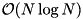, where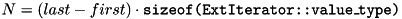.
• I/O complexity: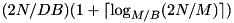I/Os

stxxl::sort chooses the block size (parameter B) equal to the block size of the container, the last and first iterators pointing to (e.g. stxxl::vector's block size).

The second term in the I/O complexity accounts for the merge phases of the external memory sorting algorithm . Avoiding multiple merge phases speeds up the sorting. In practice one should choose the block size B\$of the container to be sorted such that there is only one merge phase needed: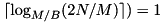. This is possible for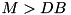and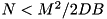. But still this restriction gives a freedom to choose a variety of blocks sizes. The study  has shown that optimal B for sorting lies in the range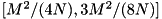. With such choice of the parameters the stxxl::sort always performs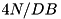I/Os.

# Internal Memory Consumption

The stxxl::sort consumes slightly more than M bytes of internal memory.

# External Memory Consumption

The stxxl::sort is not in-place. It requires about N bytes of external memory to store the sorted runs during the sorting process . After the sorting this memory is freed.

# Example

struct MyCmp: public std::less<int> // ascending order
{
static int min_value() const
static int max_value() const
};
vec_type V;
// ... fill here the vector with some values
// Sort in ascending order use 512 MiB of main memory
stxxl::sort(V.begin(), V.end(), MyCmp(), 512*1024*1024);
// sorted

# Sorted Order Checking

STXXL gives an ability to automatically check the order in the output of STXXL sorters and intermediate results of sorting (the order and a meta information in the sorted runs). The check is switched on if the source codes and the library are compiled with the option -DSTXXL_CHECK_ORDER_IN_SORTS and the option -DNDEBUG is not used. For details see the compiler.make file in the STXXL tar ball. Note, that the checking routines require more internal work as well as additional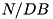I/Os to read the sorted runs. Therefore for the final non-debug version of a user application on should switch this option off.

This checker checks the stxxl::sort, stxxl::ksort, and the pipelined sorter.